# Tips To Solve Syllogism Questions

In this article, we’ll discuss about useful tips and tricks to solve Syllogism Questions for various competitive Government and bank exams.

The syllogism is a topic in the reasoning part, which is a very important section in most of the competitive exams like the Bank Exams, Railways, SSC, etc. This topic, i.e. the syllogism generally has very high weightage in the reasoning section and thus has become very important for the exams.

With respect to the latest exams that have been conducted, the syllogism questions in the reasoning section comprise 4 to 5 questions in the prelims exam. Thus, candidates must know the syllogism tricks to solve these questions.

The syllogisms are just argument sentences that require deductive reasoning to arrive at some conclusions. Presently, the SBI and IBPS exams ask the syllogism questions in a reversed way, i.e. statements are asked from the given conclusions.

There are certain syllogism tricks that will help in solving these questions easily and candidates can refer to this article to know the different syllogism tricks.Here are the different competitive exams which include the syllogism topic in its syllabus:

The bank exams syllabus includes Syllogism as a main topic of the Reasoning Ability section.

Questions based on syllogism form an important part of the reasoning section and candidates cannot skip preparing these questions as it may result in candidates solving lesser questions in the reasoning sector. Hence, candidates must refer to some syllogism tricks to solve these questions.

In this article, some examples are provided for the most common types of syllogism questions along with Venn diagrams to solve them with ease.

## Syllogism Questions

1. All A are B

This phrase simply means that A is contained in B but not necessarily vice versa. This means A is a subset of B but B may not be a subset of A. The Venn diagram for this is: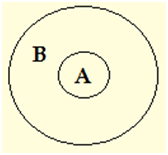In this diagram, it is clearly visible that circle A is inside the circle B which simply means that B contains the entire A i.e. All A are B.

2. A = B

In this case, the conclusion is similar to the first type i.e. “All A are B”. Here not only “All A are B”, but also “All B are A”. This means A is a subset of B and B is also a subset of A. The Venn diagram is: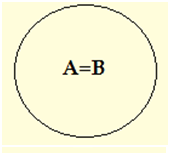Here A is contained in B and so is B contained in A. So, here A contains all B and again B also contains all A.

3. No A are B

It is simply understandable that B does not contain any of A and so A is not contained in B. This means that A and B are disjoint sets. The Venn diagram for this case is: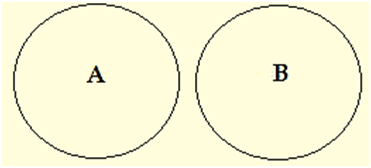Here no part of A is present inside of B and similarly, no part of A is present in A. So neither A nor B contain any part of B or A respectively.

 Important Govt Exams Related Topics SBI Exams IBPS Exams RBI Exam SSC GD Syllabus SSC CGL Syllabus SSC CPO Syllabus SSC CHSL Syllabus SSC JE Syllabus

4. Some A are B

This is the case when some of A is in B that is A and B are intersecting and thus some B are A will also be true. The Venn diagram depiction is as: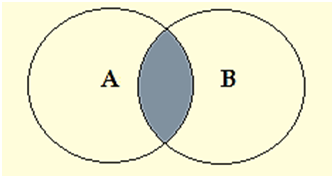Here, the shaded portion simply indicates that some portion of A is contained in B while the unshaded portion is uncertain portion and does not indicate anything whether A is contained in B or not.

5. Some A are not B

This means that some portion of A is not included in B for sure while the other part of A is uncertain whether it is included in B or not. The Venn diagram is;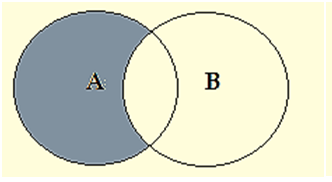In this, some portion of A is surely not included in B while there is no surety whether the shaded region is included in B or not.

These are certain universal rules that should be followed while solving the syllogism questions. They are:

1. Any “All” and “All” sentence will always imply an “All” conclusion.
2. Any “All’ and “No” sentence will always imply a “No” conclusion.
3. Any ‘All” and “Some” sentence will always imply a “No” conclusion.
4. Any “Some” and “All” sentence will always imply a “Some” conclusion.
5. Any “Some” and “No” sentence will always imply a “Some not’ conclusion.
6. Any “Some” and “Some” sentence will always imply a “No” conclusion.

For more details on Syllogism – Reasoning Questions and Problems, check at the linked article.

Tips to solve the questions related to Syllogism:

2. Start drawing the Venn diagram
3. Follow the sequence of the question while drawing
4. Analyse the conclusion from the Venn diagram
5. Check for other alternative solutions at the end

Example:

Statements:

1. Some pencils are dogs
2. All dogs are pens
3. All pens are cats

Conclusions:

1. All dogs are cats
2. Some pens are pencils
3. Some pencils are cats

Solution:

Analysing the first statement, the Venn diagram can be made as;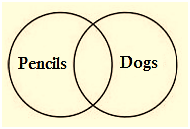Now as per the second statement, all dogs are pens, we can draw the Venn diagram as: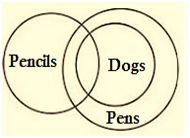Now as per the last statement which says that all pens are cats, we get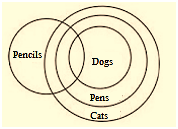This is the total representation of the statements. Now the conclusion needs to be analysed one by one.

For the first conclusion, it is seen that the circle dogs is engulfed inside the circle cats. Thus the conclusion “all dogs are cats” is true.

For the second conclusion, the circles’ pens and pencils intersect each other and hence, the conclusion “some pens are pencils” is also true.

For the third conclusion, the circles’ cats and pencils also intersect each other and hence the conclusion “some pencils are cats” is also true.

Therefore, all the conclusion in this question is true.

In this way, the questions related to syllogism can be easily solved. The only thing that is important is to practice different variations of syllogism related questions and various bank exam questions so as to build up the confidence gradually.

### Syllogism Tricks and Tips

Candidates can follow the below-mentioned syllogism tricks and tips that may help them solve sylloge questions easily:

1. Always pay attention to words like ‘some’, ‘a few’, ‘all’, ‘atleast’, etc. These words form the base to solve the syllogism questions.
2. The best syllogism trick is to solve questions in the form of Venn diagrams. This will make the explanation more clear and simplified.
3. Never assume anything while solving the syllogism questions. The only data that has to be followed while solving the question is the data mentioned in the question. No extra assumption must be made while solving questions.

Solve the examples given in this article based on the syllogism trick given above. Candidates shall find these syllogism tricks to be useful while solving the questions.

Given below are a few links where candidates can find more such syllogism questions and they can follow the syllogism tricks to solve them: